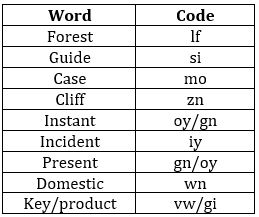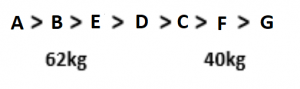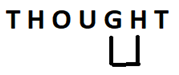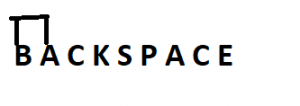Latest Banking jobs   »

# Reasoning Ability Quiz For Bank Foundation 2nd January

Directions (1-5): Study the following information carefully and answer the questions given below:
In a certain code language,
‘Forest guide case cliff’ is written as ‘mo zn si lf’,
‘Instant guide incident present’ is written as ‘gn iy oy si’,
‘Cliff case key product’ is written as ‘vw mo zn gi’,
‘Domestic case present instant’ is written as ‘gn oy mo wn’.

Q1. What is the code for ‘Domestic’?
(a) wn
(b) mo
(c) oy
(d) gn
(e) None of these

Q2. Which of the following may be the code for ‘product guide case’?
(a) vw si iy
(b) gi mo si
(c) iy si gi
(d) vw mo si
(e) Can’t be determined

Q3. What is the code for ‘Key’?
(a) gi
(b) zn
(c) mo
(d) vw
(e) Can’t be determined

Q4. What does ‘iy’ stand for?
(a) Guide
(b) Instant
(c) Incident
(d) Present
(e) None of these

Q5. What is the code for ‘Case’?
(a) zn
(b) si
(c) oy
(d) mo
(e) Can’t be determined

Q6. The position of how many alphabets will remain unchanged if each of the alphabets in the word ‘INTERVATION’ is arranged in alphabetical order from left to right?
(a) Three
(b) None
(c) One
(d) Two
(e) None of these

Q7. In an exam, A, B, C and D each scored different marks. B scored more than C and D. No one scored less than A. Who amongst them scored the maximum marks?
(a) B
(b) Either C or D
(c) D
(d) Cannot be determined
(e) None of these

Q8. In a row, Rajiv is twenty-first from the left end and Shivani is fifteenth from the right end. If they interchange their positions Shivani is twenty-seventh from the right end. How many people are there in the row?
(a) 46
(b) 47
(c) 56
(d) 59
(e) None of the above

Q9. If it is possible to make only one meaningful word with the second, fourth, sixth and eighth letters of the word SERVICEABLE, which of the following will be the first letter of that word? If only two such words can be formed, give ‘X’ as the answer; if three or more such words can be formed, give ‘Y’ as your answer and if no such word can be formed, give ‘Z’ as the answer.
(a) C
(b) A
(c) X
(d) Z
(e) Y

Direction (10-12): Study the following information carefully and answer the given questions.
There are seven friends i.e. A, B, C, D, E, F and G having different weights. At least three persons are heavier than D. B is not the heaviest. C is heavier than F but lighter than D. G is the lightest. B is heavier than D and E. The weight of second lightest person is 40 kg and second heaviest person is 62 kg.

Q10. If the weight of D is 52kg, then what may be possible weight of C?
(a) 56kg
(b) 70 kg
(c) 48kg
(d) 35 kg
(e) None of the above

Q11. How many persons are lighter than E?
(a) One
(b) None
(c) Three
(d) Two
(e) Four

Q12. Who is just heavier than E?
(a) D
(b) A
(c) B
(d) F
(e) None of these

Q13. How many such pairs of letters are there in the word ‘THOUGHT’ each of which has as many letters between them in the word as in the English alphabet (Both forward and backward)?
(a) One
(b) None
(c) Three
(d) Two
(e) Four

Q14. If each of the vowels in the word WEQIL is kept unchanged and each of the consonants is replaced by the next letter in English alphabet, how many meaningful words can be formed with the new letters using each letter only once in each word?
(a) One
(b) None
(c) Three
(d) Two
(e) Four

Q15. How many such pairs of letters are there in the word ‘BACKSPACE’ each of which has as many letters between them in the word as in the English alphabet (Both forward and backward)?
(a) One
(b) None
(c) Three
(d) Two
(e) More than three

Solutions

Solution (1-5):
Sol.S1. Ans. (a)
S2. Ans. (e)
S3. Ans. (e)
S4. Ans. (c)
S5. Ans. (d)

S6. Ans. (b)
Sol. Given Word- INTERVATION
After Arrangement- AEIINNORTTV

S7. Ans. (a)
Sol. B scored more than C, D or A.

S8. Ans. (b)
Sol. So, Total number of students = Rajiv position from left + Shivani position from right rearrangement – 1. So, 27 + 21 – 1 = 47

S9. Ans. (a)
Sol. 2nd, 4th, 6th and 8th letters = E, V, C, A
Meaningful word = CAVE.

Solutions (10-12):
Sol.S10. Ans. (c)
S11. Ans. (e)
S12. Ans. (c)

S13. Ans. (a)
Sol.S14. Ans. (d)
Sol. New word = XERIM
Meaningful words using X, E, R, I and M = Remix, Mixer.

S15. Ans. (a)
Sol.#### Congratulations!Union Budget 2023-24: Free PDF